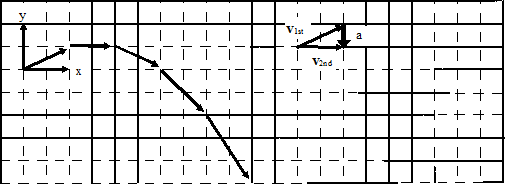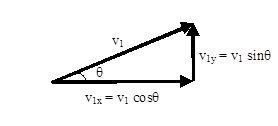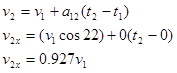$$\require{cancel}$$

# 04. Tabulating Motion Information & Doing The Math

•• Contributed by Paul D'Alessandris
• Professor (Engineering Science and Physics) at Monroe Community College

# Tabulating Motion Information

In the shot put, a large mass is thrown at an angle of 22oabove horizontal, from a position of 2 m above the ground, a horizontal distance of 25 m.Now that you have constructed a motion diagram and motion graphs, you should be able to assign numerical values to several of the kinematic variables. A glance at the situation description should indicate that information is presented about the shot put at two distinct events: when the shot put leaves the putter’s hand and when the shot put strikes the ground. Other information can also be determined about these events by referencing the motion diagram. To organize this information, you should construct a motion table.In addition to the information explicitly given (the initial and final positions), information is available about both the initial velocity and the acceleration.

• Initial velocity: Although the magnitude of the initial velocity (v1) is unknown, its orientation in space is known. Thus, via the right-angle trigonometry shown below, the components of this unknown magnitude velocity in the horizontal and vertical directions can be determined. Since we will analyze the x- and y-motion separately, we mustbreak the initial velocity into its x- and y-components.• Acceleration: The only force acting on the shot-put during the time interval of interest is the force of gravity, which acts directly downward. This is because the analysis of the motion is restricted to the time interval after leaving the thrower’s hand and before striking the ground. Thus, there is no horizontal acceleration of the shot-put and the vertical acceleration has a magnitude of 9.8 m/s2.

# Doing the Math

In Model 1, you were presented with two kinematic relationships. These relationships are valid in both the horizontal and vertical directions. Thus, you have a total of four relationships with which to analyze the scenario given. In the example above, there are four unknown kinematic variables. You should remember from algebra that four equations are sufficient to calculate four unknowns. Thus, by applying the kinematic relations in both the horizontal and vertical directions, you should be able to determine the initial velocity of the shot-put, the time in the air, and the final horizontal and vertical velocity components.

First, let’s examine the horizontal component of the motion. Note that the positions, velocities, and accelerations in the following equations are all horizontal components.

x-directionNow let’s examine the vertical component of the motion. All the positions, velocities, and accelerations in the following equations are now vertical components.

y-directionSubstituting the value of v1from above yields:Plugging t2= 1.57 s into all of the remaining equations gives:

v1 = 17.2 m/s v2x = 15.9 m/s v2y= -8.94 m/s